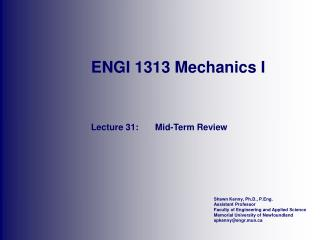# ENGI 1313 Mechanics I - PowerPoint PPT PresentationDownload PresentationENGI 1313 Mechanics I

ENGI 1313 Mechanics I
Download Presentation## ENGI 1313 Mechanics I

- - - - - - - - - - - - - - - - - - - - - - - - - - - E N D - - - - - - - - - - - - - - - - - - - - - - - - - - -
##### Presentation Transcript

1. ENGI 1313 Mechanics I Lecture 31: Mid-Term Review

2. Overall Mid-Term Results • Class Statistics (259) • Average • 67% • Standard deviation • 17% • Standard deviation between sections • 3% • Solution Posted

3. Term A/B Exam (Mon. Oct 22) • Results are not Back • Student Numbers • 200600816 • 200641421 • 200641728 • 200643385 • 200714459 • 200737914 • 200749075

4. No Record of Mid-Term Exam • Contact Me Immediately to Resolve • Student Numbers • 200643849 • 200626752 • 200402782 • 200565687 • 200672285

5. Problem 1 • Determine the components of the F force acting along the u and v axes. • Given: • 1 = 70 • 2 = 45 • 3 = 60 • F = 250N

6. Problem 1 (cont.) • Problem Characteristics • Force vectors • No right rectangular coordinate system • Forces on specified axes • Solution Method • Parallelogram or triangle construction • Law of sines

7. Problem 1 (cont.) • Force Triangle  Fv F = 250 N 2 = 45 1 = 70 Fu

8. Problem 2 • The three cables are used to support the lamp of weight W. Determine the force developed in each cable for equilibrium. • Given: • a = 4 m • b = 4 m • c = 2 m • W = 600 N 4 m 2 m 4 m W = 600 N

9. Problem 2 (cont.) • Problem Characteristics • Particle Equilibrium • Scalar or vector approach • Solution Method • Summation of Forces 4 m 2 m 4 m W = 600 N

10. Problem 2 (cont.) • FBD and Unit Vectors FAD 4 m 2 m 4 m FAB FAC W = 600 N

11. Problem 2 (cont.) • Equilibrium Equation • Three Equations FAD 4 m 2 m 4 m FAB FAC W = 600 N

12. Problem 3 • Force Fis applied to the handle of the wrench. Determine the angle  between the tail of the force Fand the handle AB. • Given: • a = 0.30 m • b = 0.50 m • F = 80 N • 1 = 30 • 2 = 45 F = 80N 1  2 0.3 m 0.5 m

13. Problem 3 (cont.) • Problem Characteristics • Vector projection • Solution Method • Dot product F = 80N 1  2 0.3 m 0.5 m

14. Problem 3 (cont.) • Unit Vectors • Dot Product F = 80N 30  45 0.3 m 0.5 m

15. Problem 4 • The hood of the automobile is supported by the strut AB, which exerts a force F on the hood. Determine the moment of this force about the hinged axis y. • Given: • a = 0.60 m • b = 1.50 m • c = 0.25 m • d = 1.00 m • F = 100 N

16. Problem 4 (cont.) • Problem Characteristics • Moment about aspecified axis • Solution Method • Triple scalar product

17. Problem 4 (cont.) • Unit Vector • Moment about Y-axis